Computer Science

# Practice with event streams

These problems will give you a chance to practice using event streams to solve problems. The idea behind this pattern is that we use a `while` loop to move through the world, and then inside the while loop we use an `if` statement to modify the world, depending on some conditions:

``````while keep_moving(bit):
if some_condition(bit):
do_something(bit)
elif some_other_condition(bit):
do_another_thing(bit)
else:
do_the_default_thing(bit)``````

As you work on these problems, remember to:

• Break your solution into multiple pieces, with each one being a function.
• Write and test one function as a time.
• As you write a function, define the stopping conditions and use the event stream pattern.
• Use glue code in between functions as needed.

When you are debugging, you can use `bit.snapshot()` to capture what the world looks like at that point, and then use buttons to jump to snapshots.

Download the zip file above and put it in your `bit` folder.

## Hurdles

For this problem, Bit needs to jump hurldes. Here is an example world:To complete the task, Bit needs to walk over each hurdle, with its path painted in green: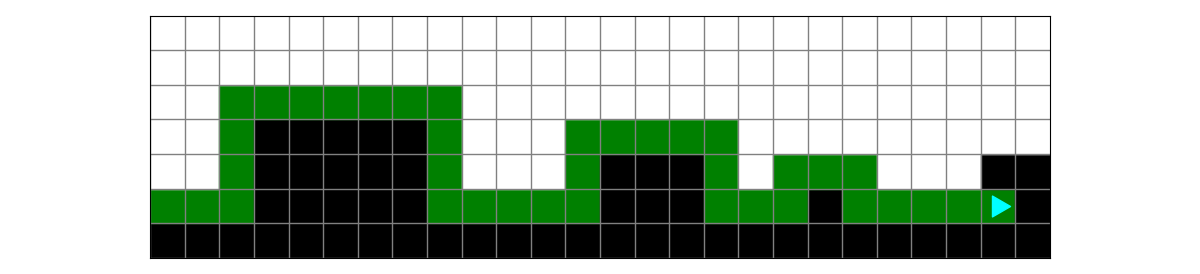Be aware! There is a second world that looks like this:and the finish looks like this:This makes solving the problem a little tricky! Bit might start at a hurdle and there may not be much space between hurdles.

### Planning

Draw out a solution to this problem. Think about how you would get Bit to move forward and walk any hurdles it encounters. What keeps Bit moving forward? What events should it handle as it moves forward?One example of what you could draw: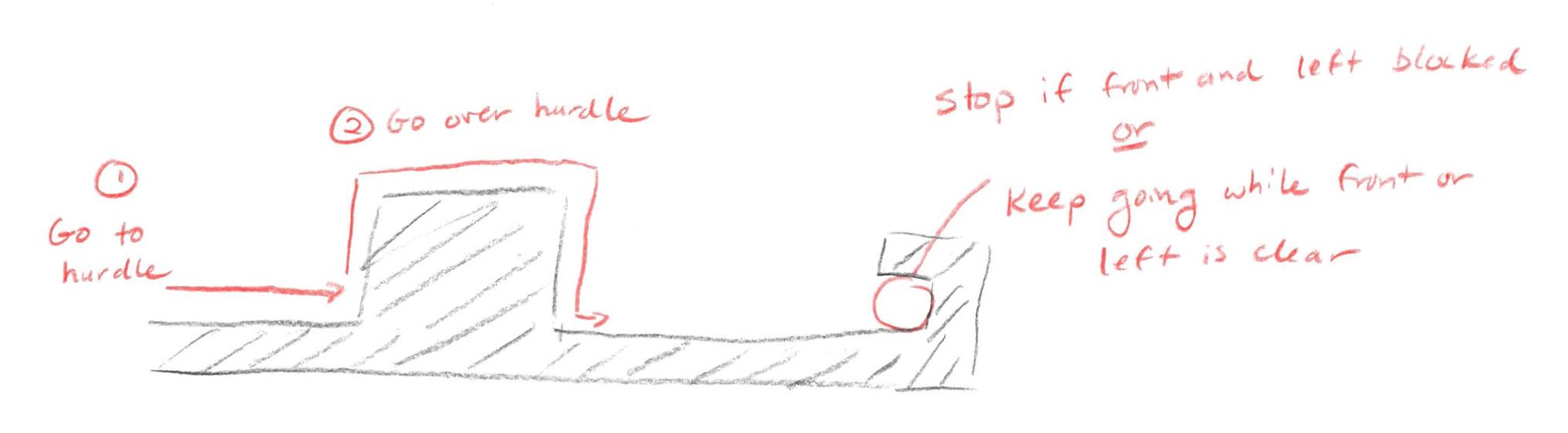This drawing is saying that there are these steps:

• move and paint until Bit gets to a hurdle
• if left is clear, go over the hurdle
• keep going until the front and left are blocked (or keep going while the front or left is clear)

In this solution, we assume there are hurdles, so we think of the solution as: move to a hurdle, go over a hurdle, and then keep doing that. In this case, we can tell Bit to keep moving or jumping hurdles as long as the front or the left is clear.

This kind of solution can work, but it is quite a bit more work to program correctly. A better way to think about it is to use the `event stream` pattern: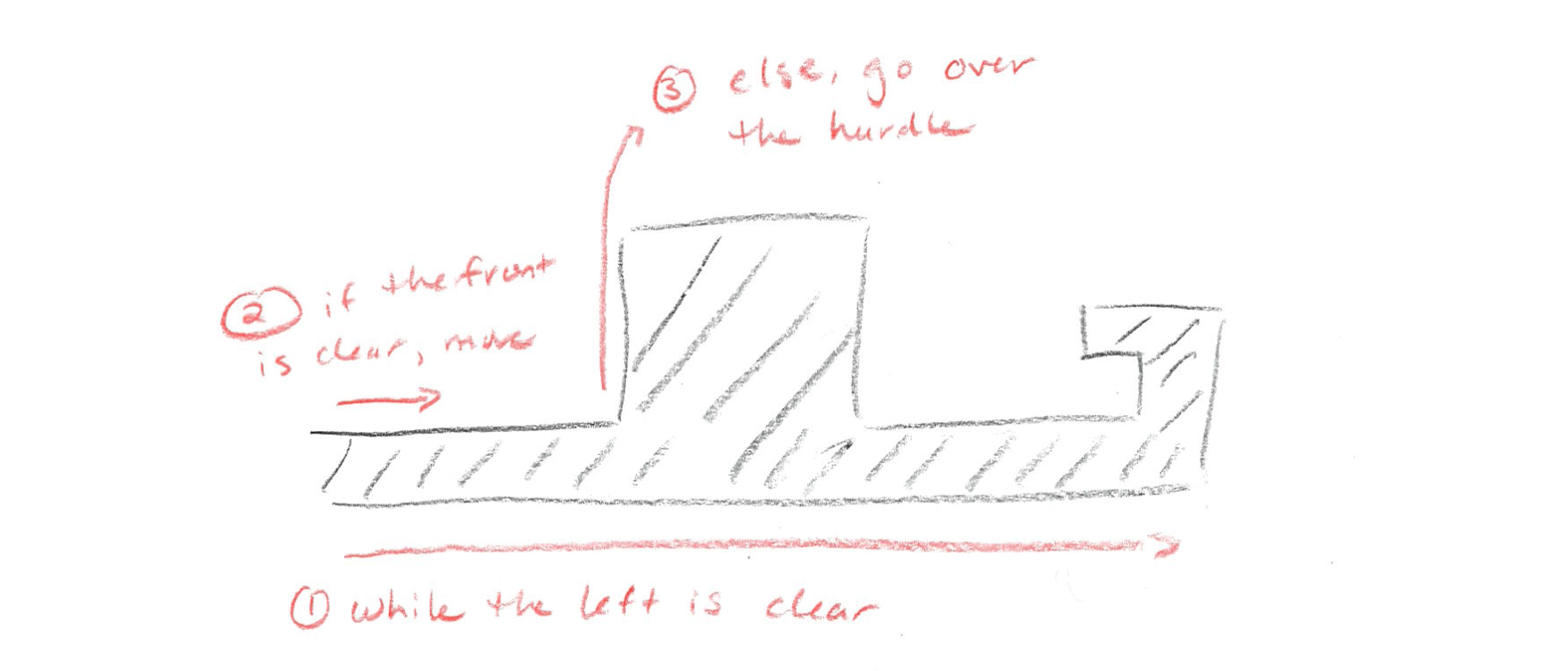• while the left is clear:
• if the front is clear:
• move and paint
• else
• go over the hurdle

In this solution, Bit keeps moving or jumping hurdles as long as the left is clear. Then inside the loop Bit moves and paints if the front is clear, otherwise (the front is not clear) it jumps over a hurdle.

The key is recognizing that Bit keeps moving as long as the left is clear (since the front won’t always be clear). Each of the worlds has the left blocked at the end.

### Starting to code

Let’s turn the second drawing into some code. In the zip file above you will find `hurdles.py`:

``````from byubit import Bit

@Bit.worlds('hurdles', 'more-hurdles')
def run(bit):
# Write code here
pass

if __name__ == '__main__':
run(Bit.new_bit)``````

In the run function, we can write the event stream:

``````def run(bit):
bit.paint('green')
while bit.left_clear():
if bit.front_clear():
bit.move()
bit.paint('green')
else:
go_over_hurdle(bit)``````

Write an empty `go_over_hurdle()` function:

``````def go_over_hurdle(bit):
pass``````

Run this code and see how we are doing so far: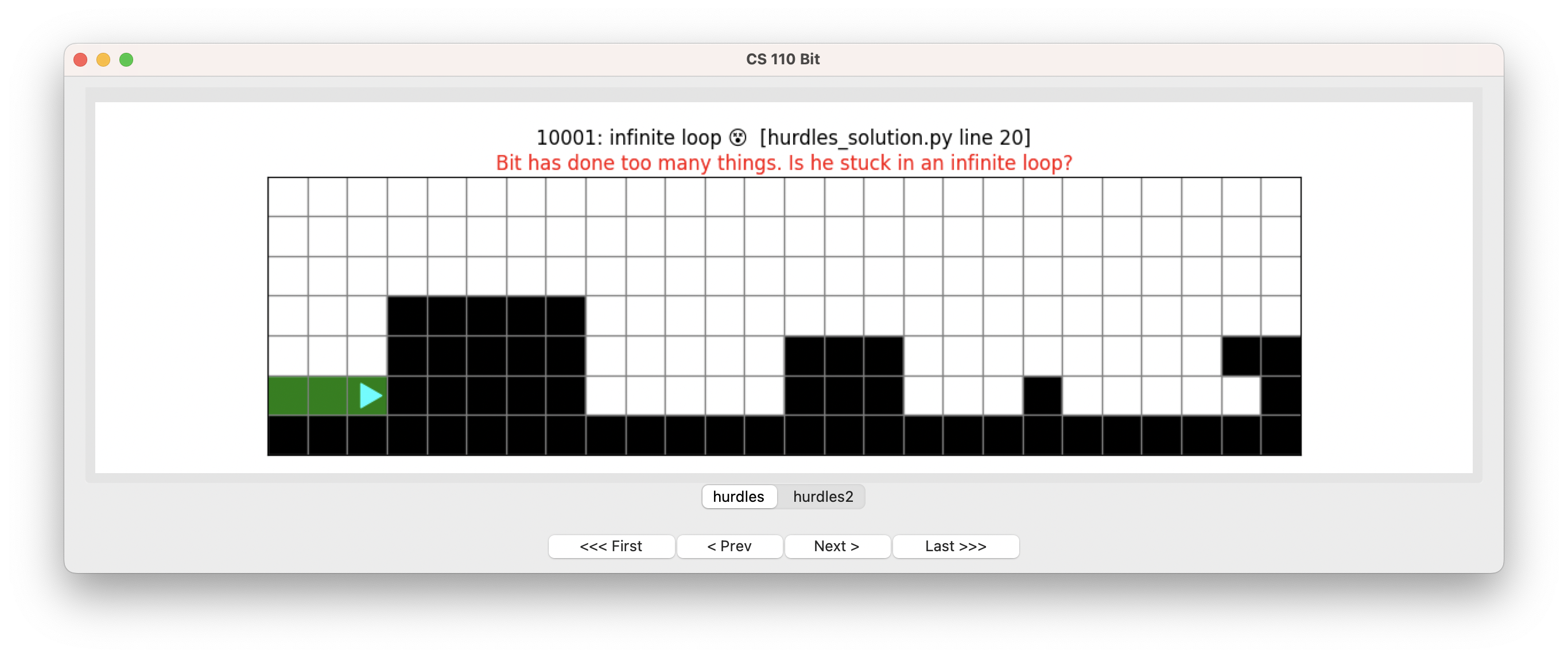Do not be discouraged! In this case, Bit gets into an infinite loop. This is because we told Bit to keep moving as long as the left is free, but left out going over hurdles. So Bit gets to a hurdle and does nothing, but the left is still free.

Here is the second world:Not much is happening in the second world because Bit starts at a hurdle. But we did paint the first square green. :-)

### Going over a hurdle

Now let’s do the part where Bit goes over a hurdle. It helps to again think of this as an event stream:If we turn Bit left, then what is the stopping condition? We want to keep going as long as the front is clear. As we are doing this, if the right is clear, then Bit should turn right.

``````def go_over_hurdle(bit):
# turn left
bit.left()
# an event stream for the hurdle
while bit.front_clear():
bit.move()
bit.paint('green')
if bit.right_clear():
bit.right()

# turn left again
bit.left()``````

Run this code now and see if we can make it over the hurdles: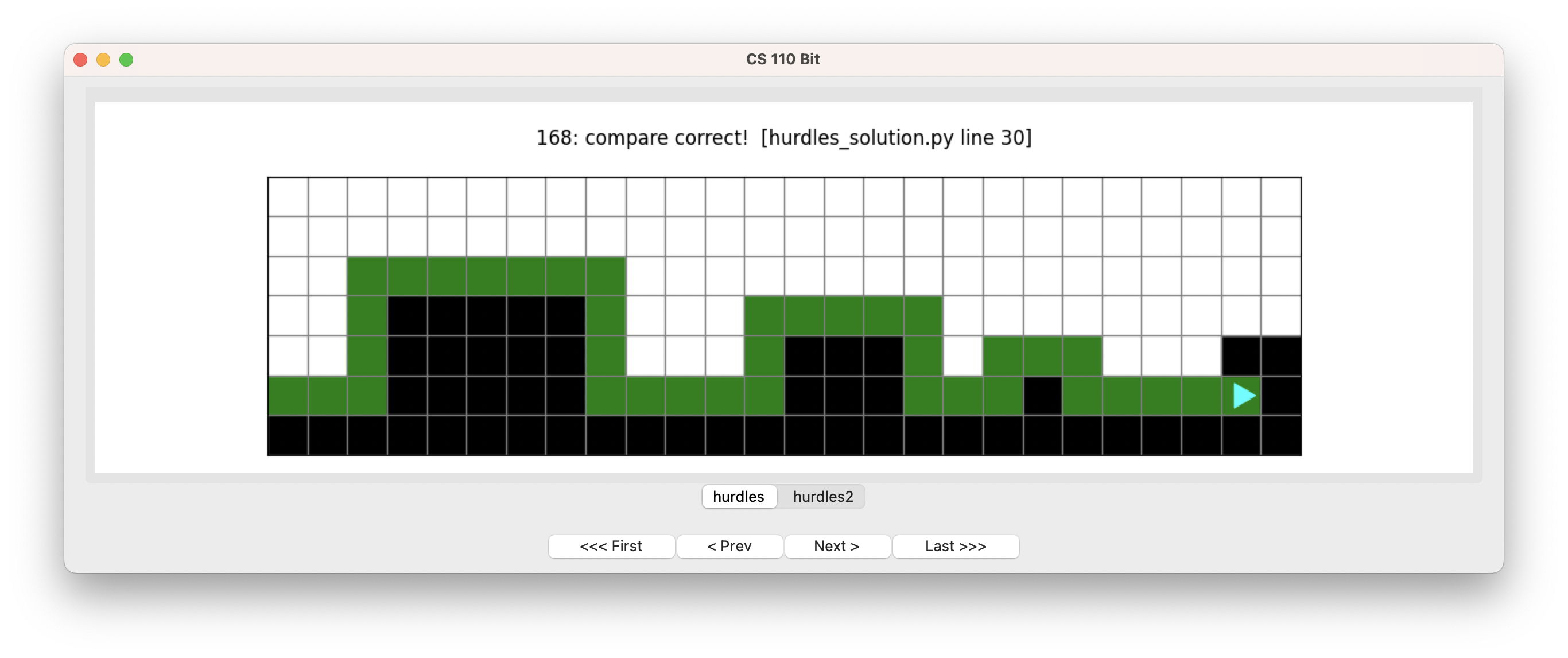Excellent!

Let’s also check that it solves the second world: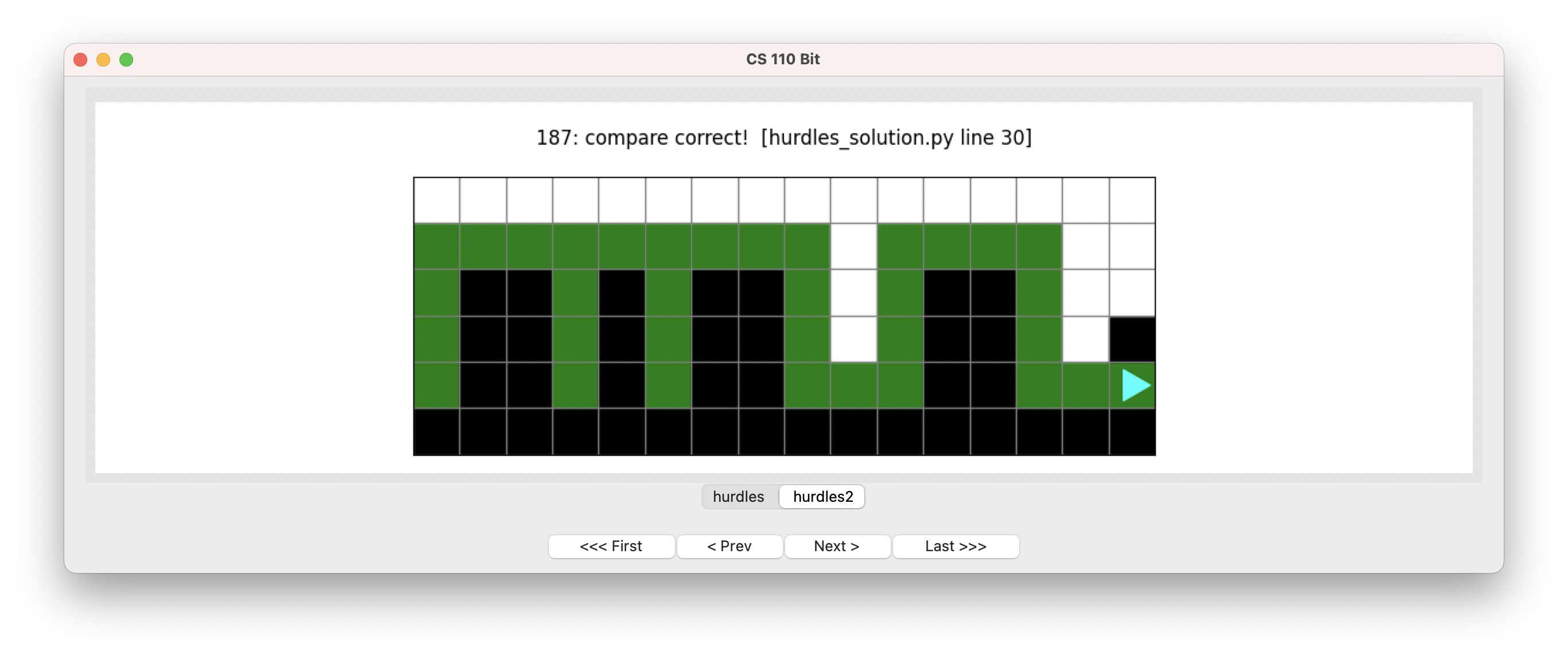Stupendous!

## Elevators

In this problem, Bit needs to take a series of elevators. The world looks like this: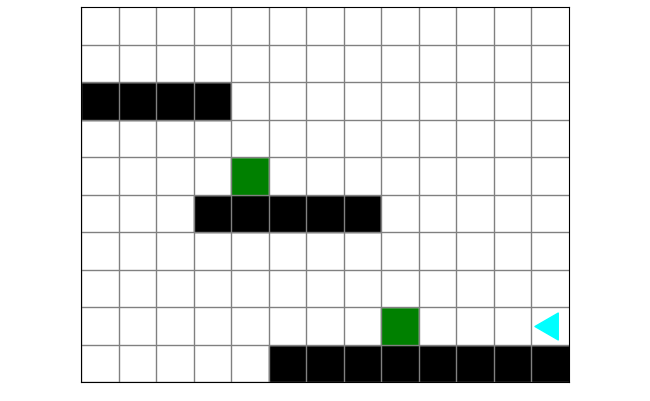The floors are in black and the elevators are represented with green. Bit travels from right to left and, when it meets an elevator, goes up to the next floor. At the end, the world should look like this:### Planning

Draw out a solution to this problem. What steps does Bit need to take? What is the stopping condition?Here is one way to draw this out:• while the front is clear
• move forward
• if the square is green
• go up

### Writing the code

Let’s turn this drawing into some code. In the zip file above you will find `elevators.py`:

``````from byubit import Bit

@Bit.worlds('elevators')
def run(bit):
# Write code here
pass

if __name__ == '__main__':
run(Bit.new_bit)
``````

We can put the main loop for the event stream pattern into the `run()` function:

``````def run(bit):
while bit.front_clear():
bit.move()
if bit.is_green():
go_up(bit)``````

We can then define `go_up()`:

``````def go_up(bit):
# turn right
bit.right()
# go up the elevator
while bit.left_clear():
bit.move()
bit.paint('green')

# go above the floor and then turn left
bit.move()
bit.left()``````

If we run this code, here is what happens: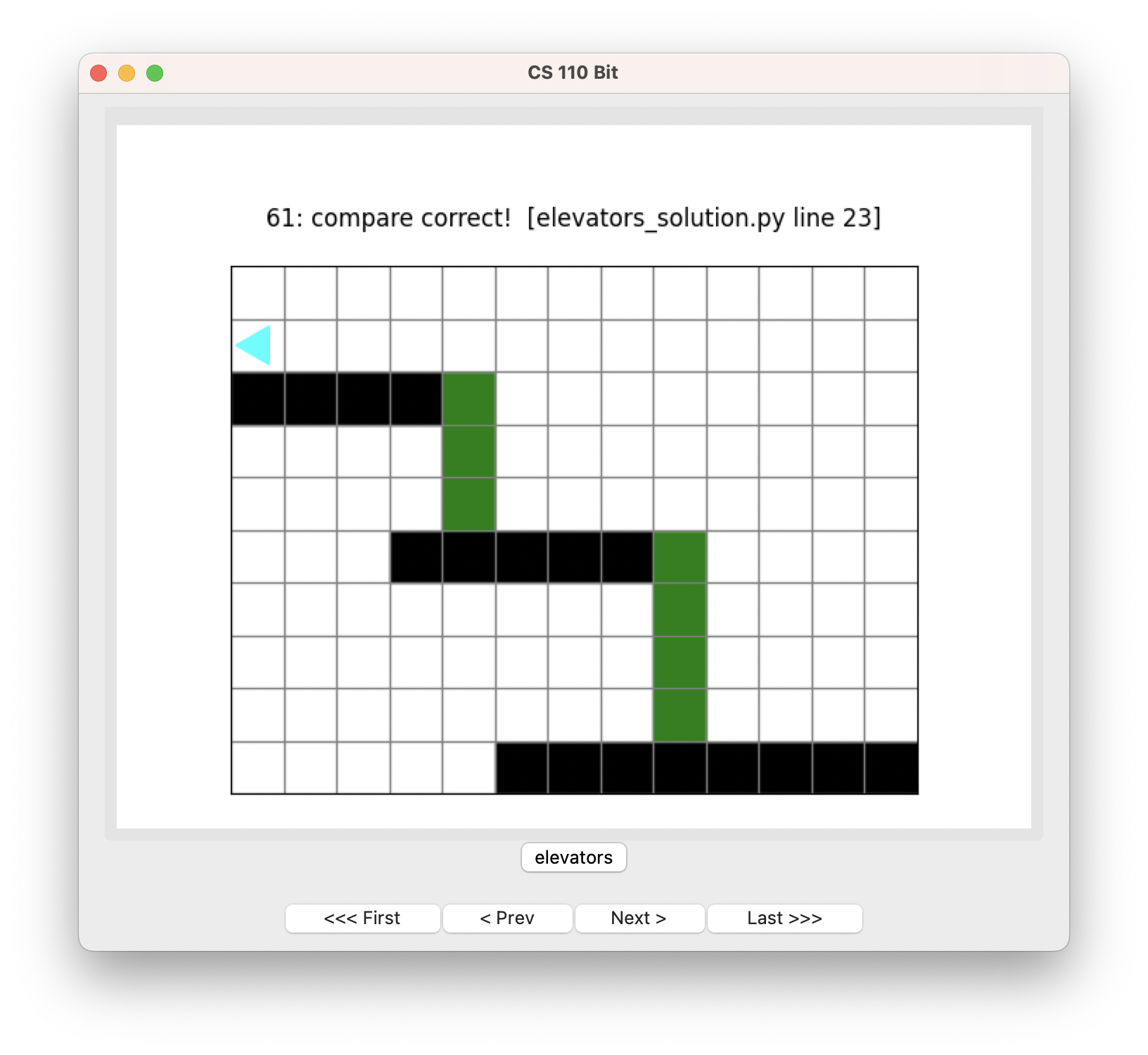Nice! Use the buttons to step through the code to be sure you understand how it works.